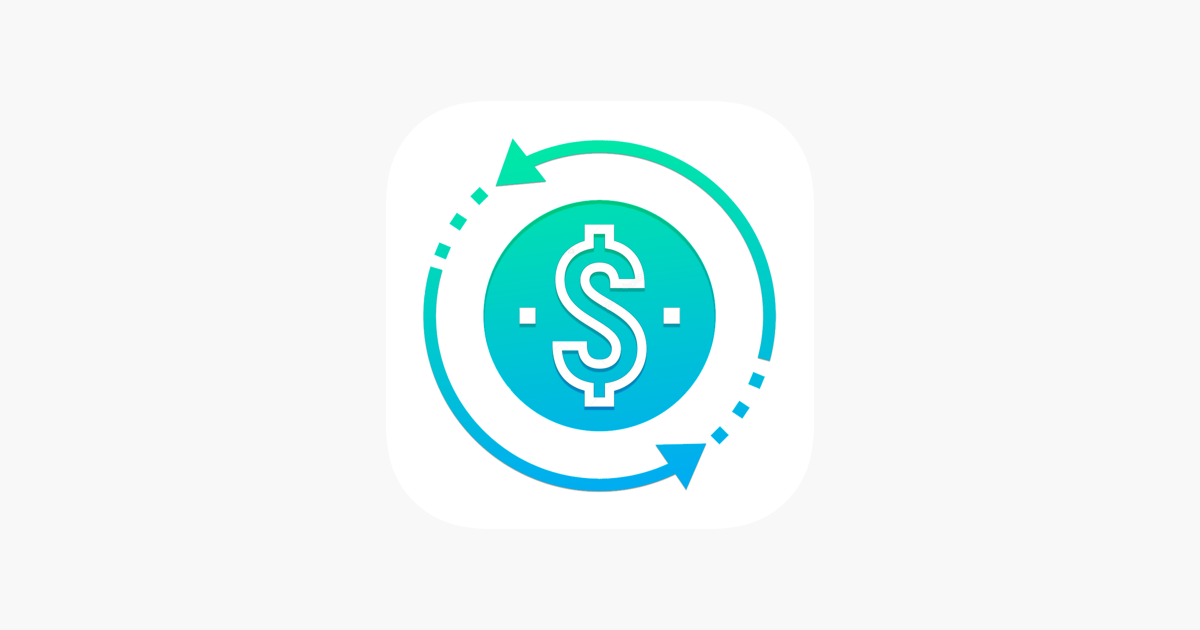# Good Contribution – murtaza vanthaliCalculate the annual interest on the deposit or the time to multiply your deposit to the desired amount in the Good Contribution mobile application. Suppose you have a certain amount that you want to multiply by annual interest and you know how much you want to receive in 5 years, then the application will help you find out the exact required annual interest for this. Or if you want to know the number of years you need to get a certain amount at a certain percentage. Install Good Contribution and calculate financial formulas quickly and easily!